Function Repository Resource:

ChaosGame

Plot iterations for the 2D chaos game

Contributed by: Eric Weisstein
 ResourceFunction["ChaosGame"][n,k,d] gives k iterations of the 2D chaos game on a regular n-gon with iter points using a ratio of 1/2, dropping the first d points. ResourceFunction["ChaosGame"][{n,r},k,d] uses a ratio r.

Details

In a chaos game, one picks a point at random inside a regular n-gon, and then draws the next point a fraction r of the distance between it and a polygon vertex picked at random. The process is continued for k iterations (after throwing out the first d points).

Examples

Basic Examples (1)

Get three iterations:

 In:=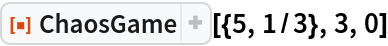Out=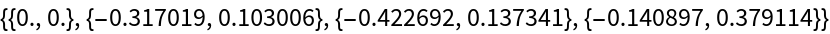Scope (2)

Try several geometries:

 In:=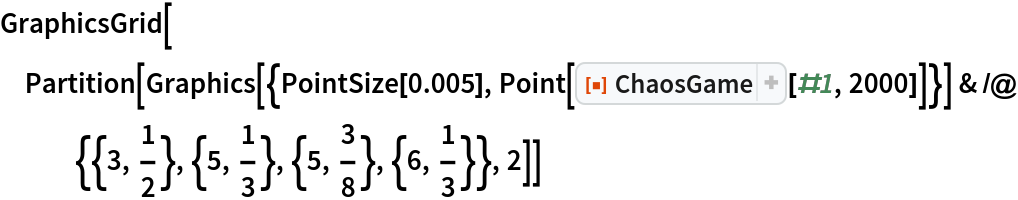Out=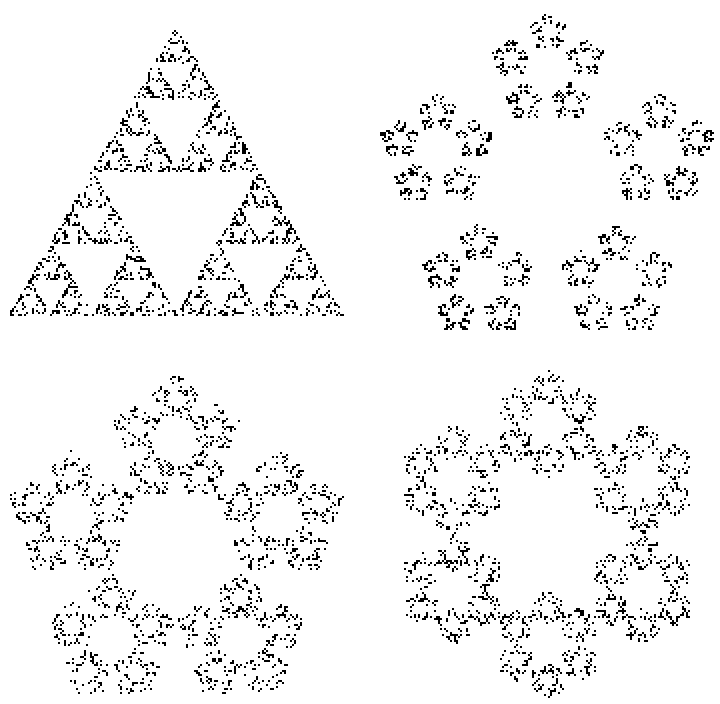Use more iterations:

 In:=Out=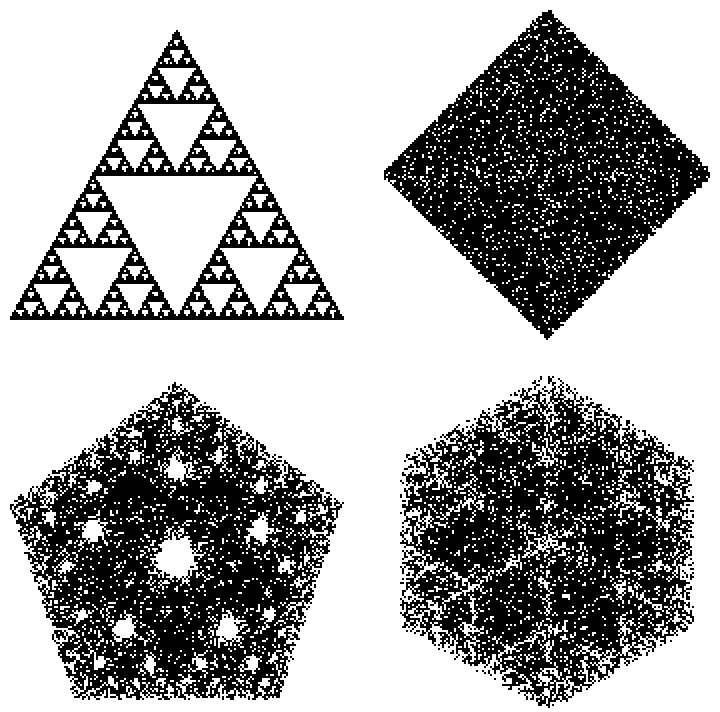Increase ratios:

 In:=Out=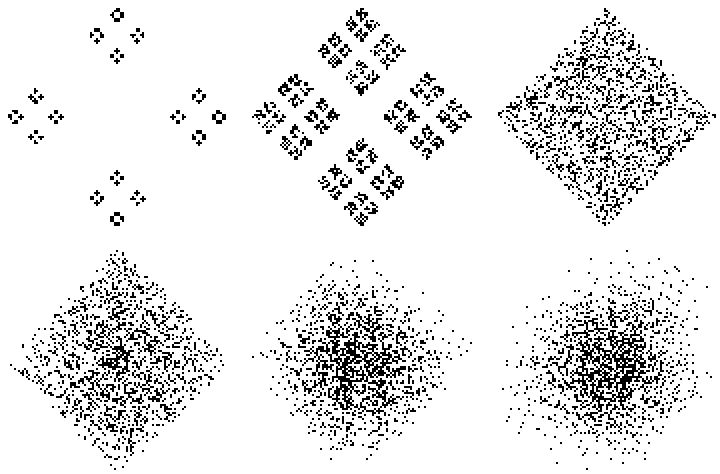Neat Examples (1)

An animation of the process:

 In:=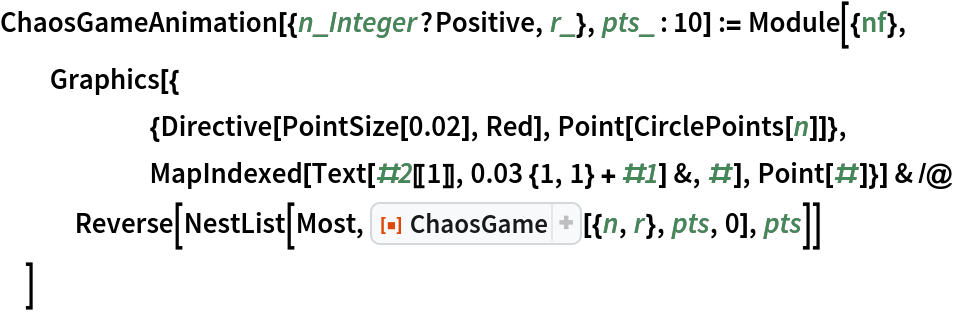In:=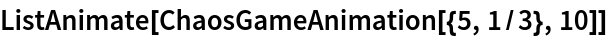Out=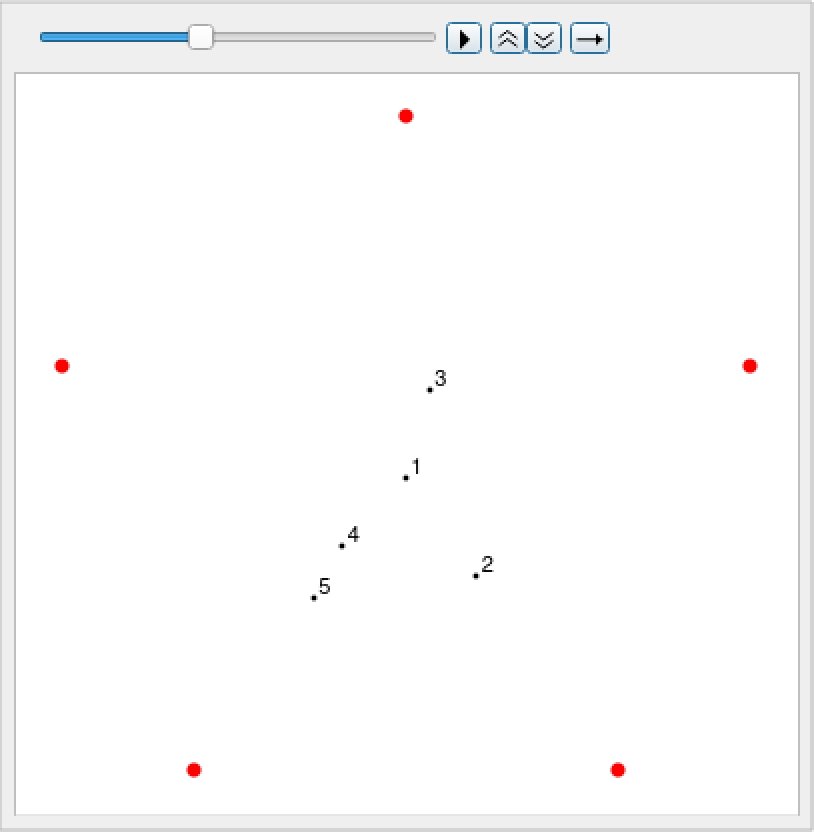Requirements

Wolfram Language 11.3 (March 2018) or above